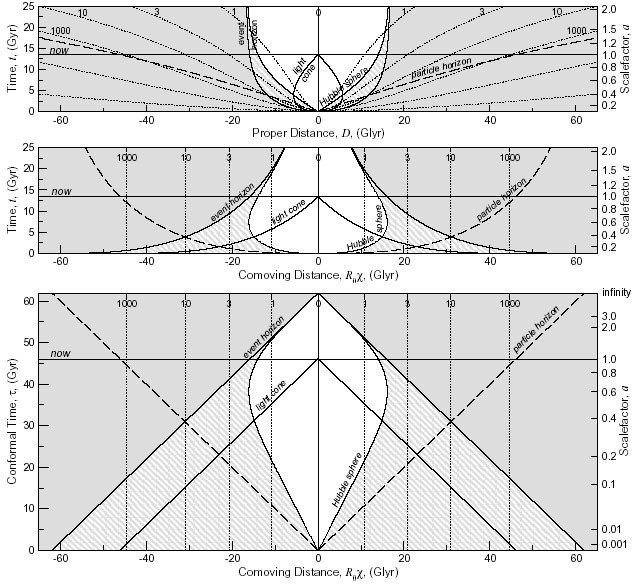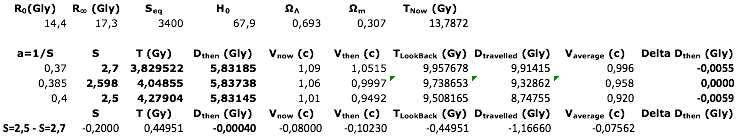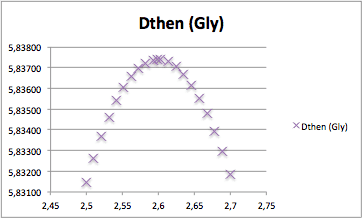# Physical meaning of max. proper distance at time of emisson

AlephClo
I cannot picture the physical meaning of the maximum proper distance at time of emisson. In the benchmark comological model the plot of (H/c) * (Proper distance at time of emission) versus z (the redshift) shows a maximum at z=1.6 for a proper distance of emission = 1800 Mpc (mega parsecs).

I know where the relation comes from, but cannot see its physical meaning.

Thank you

Early-on, the expansion was so fast that photons that the photons we see now that were traveling in our direction were actually receding. Since the proper distance between our location and the photon increased for a time, its source was actually closer to us than sources that emitted later where the photon only ever moved towards us.

AlephClo
Just to make sure I get it right. For 2 photons (photon-1 z=1.59 and photon-2 z=1.61) with the same proper distance at emission time, but with small delta z (0.01) relative to the maximum (z= 1.6), the one with z=1.59 would have to travel more distance for the extra 0.01 due to faster Universe expansion at that time, than the extra distance due to the extra 0.01 for the photon with 1.61 because Universe expansion was not as sever at that later time.

Thank you AlphaClo

It's the other way around.

First, for the distance a photon has traveled, you can trivially use the light travel time, which is by definition equal to the distance along the path the photon traveled. So a higher-redshift photon will always travel a larger distance, because light travel time does monotonically increase with redshift. So the z=1.61 photon is guaranteed to travel a longer distance.

Now, a caveat: I'm not certain that the fact that photons at high redshifts recede from us before moving toward us later changes over at exactly the same redshift that is also the maximum distance. This is a conceptual tool that can be used to understand what's going on, but it isn't necessarily exact. I would have to look into it in a bit more detail to be sure.

AlephClo
Thank you Chalnoth.
I agree that the photon with 1.61 will travel more than the 1.59. Sorry I did not expressed myself clearly.
Using a matter-lambda-no curvature model where the time of emission = 13.5Gyears * (1+z) to the -3/2
the travel time only for z = 0.01 gives:
1) for the photon-1 z=1.59 a travel time of 0.196 Gyr
2) for the photon-1 z-1.61 a travel time of 0.177 Gyr

Obviously photon-2 has to travel an extra 0.02 (1.61 - 1.59) than photon-1.

Thank you AlephClo

Incidentally, I went ahead and calculated it, and at z=1.6, the recession velocity is only about one third the speed of light, so photons receding aren't the whole of it. It works visually for very far-away galaxies, but isn't the cause right around z=1.6.

Gold Member
Dearly Missed
...I'm not certain that the fact that photons at high redshifts recede from us before moving toward us later changes over at exactly the same redshift that is also the maximum distance. This is a conceptual tool that can be used to understand what's going on, but it isn't necessarily exact. I would have to look into it in a bit more detail to be sure.
I think the changeover between photon losing ground (proper distance increasing) and approaching (distance decreasing) happens the redshift (about 1.6) where you also get the maximum proper distance at emission. That distance is about 5.8 Gly.
Incidentally, I went ahead and calculated it, and at z=1.6, the recession velocity is only about one third the speed of light, so photons receding aren't the whole of it. It works visually for very far-away galaxies, but isn't the cause right around z=1.6.
You may have miscalculated, Chal. For an object we see at z = 1.6, the recession speed at the time of emission is c, . That's why the light emitted in our direction is stalled between losing and gaining ground. At the time it is emitted, it just barely manages to hold its ground.
$${\scriptsize\begin{array}{|c|c|c|c|c|c|}\hline R_{0} (Gly) & R_{\infty} (Gly) & S_{eq} & H_{0} & \Omega_\Lambda & \Omega_m\\ \hline 14.4&17.3&3400&67.9&0.693&0.307\\ \hline \end{array}}$$ $${\scriptsize\begin{array}{|r|r|r|r|r|r|r|r|r|r|r|r|r|r|r|r|} \hline a=1/S&S&T (Gy)&R (Gly)&D_{now} (Gly)&D_{then}(Gly)&D_{hor}(Gly)&V_{now} (c)&V_{then} (c) \\ \hline 0.385&2.600&4.0442&5.8333&15.177&5.837&12.179&1.05&1.00\\ \hline 0.423&2.363&4.6381&6.6088&13.706&5.800&12.777&0.95&0.88\\ \hline 0.466&2.148&5.3092&7.4492&12.195&5.678&13.355&0.85&0.76\\ \hline 0.512&1.952&6.0634&8.3444&10.652&5.457&13.903&0.74&0.65\\ \hline 0.564&1.774&6.9052&9.2789&9.086&5.121&14.414&0.63&0.55\\ \hline 0.620&1.612&7.8372&10.2316&7.510&4.658&14.882&0.52&0.46\\ \hline 0.682&1.466&8.8602&11.1774&5.938&4.052&15.302&0.41&0.36\\ \hline 0.751&1.332&9.9721&12.0895&4.385&3.292&15.670&0.30&0.27\\ \hline 0.826&1.211&11.1686&12.9430&2.866&2.367&15.986&0.20&0.18\\ \hline 0.909&1.100&12.4430&13.7175&1.395&1.268&16.253&0.10&0.09\\ \hline 1.000&1.000&13.7872&14.3999&0.000&0.000&16.472&0.00&0.00\\ \hline \end{array}}$$
http://www.einsteins-theory-of-relativity-4engineers.com/LightCone7/LightCone.html

Last edited:
Gold Member
Dearly Missed
AlephClo,
Lightcone calculator calls the proper distance at time of emission the "distance then" or Dthen
The maximum proper distance at time of emission is about 5.8 Gly and the time of emission, you can see, is around year 4 billion. You can say the light travel time was therefore around 9.7 billion years.

A good way to picture the teardrop shape of the lightcone with its maximum bulge, its maximum girth, at z = 1.6 is to look at the TOP PANEL
of Lineweaver Figure 1. The widest point in the lightcone comes just exactly where the Hubble radius crosses it. Hubble radius is where recession speed equals c. You may need to enlarge the top panel to see that the "hubble sphere" line crosses the lightcone curve exactly where the latter is vertical---the light cone's widest point. The lightcone curve shows the career of a photon that is trying to reach us and finally does, at the present time. If it starts earlier (before year 4 billion) it is at first swept back by expanding distance, until it reaches proper distance 5.8 Gly and then it begins to make headway towards us.Last edited:
AlephClo
I have got an answer to my original. I will go over the new info tomorrow. Lost to digest.
Big thank you to both of you Marcus and Chalnoth.

You may have miscalculated, Chal. For an object we see at z = 1.6, the recession speed at the time of emission is c, . That's why the light emitted in our direction is stalled between losing and gaining ground. At the time it is emitted, it just barely manages to hold its ground.
You're right, I did miscalculate! This makes more sense now. I divided instead of multiplying.

AlephClo
Wow :-) I have purchased the book Relativity 4 Eng. and learned to use the Cosmological Calculator. Here is a summary of understanding for the records on the initial question "what is the physical meaning of 2 identical Proper Distance at Emission time?". I have worked out calculations and a graph below.
Essentially, for photons at Dthen = 5,831, one was emitted at T=3.83 Gy and observed with S=2.7 while the other photon was emitted at T=4.28 Gy and observed with S= 2.5. The bottom line gives the difference between photon S=2.5 minus photon S=2.7. The middle line is about S=2.6 where a photon would receed at about the speed of light; that is what the plot shows with more details. Thank you again for your valuable inputs. AlephClo